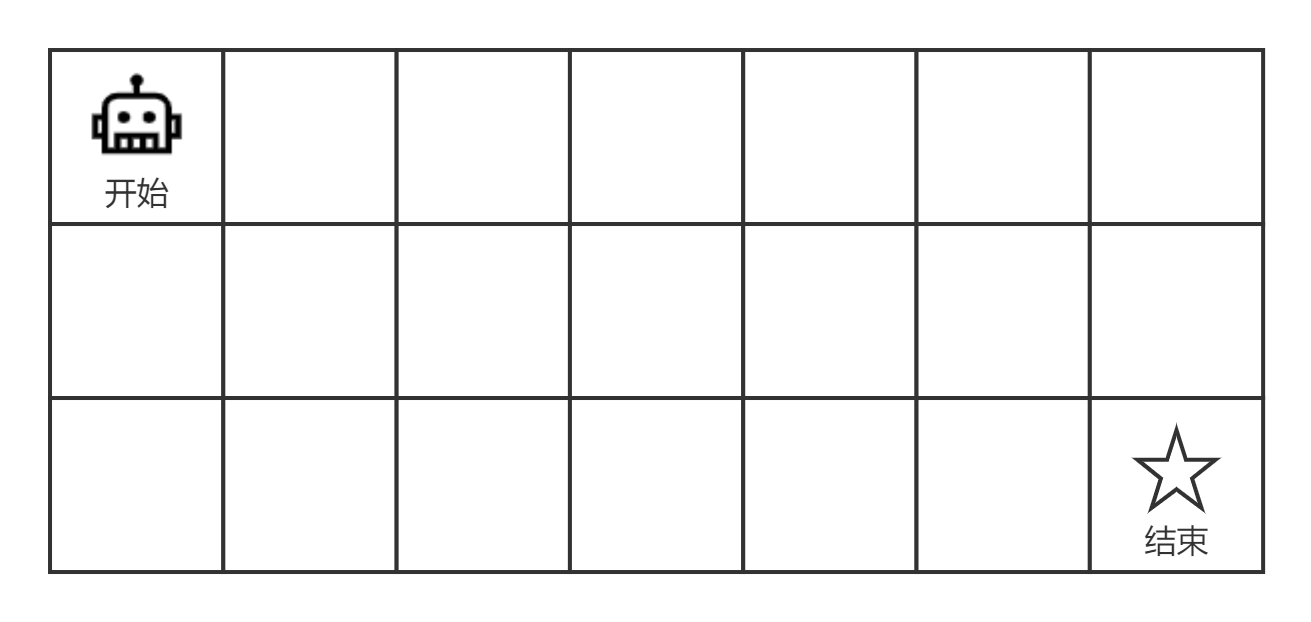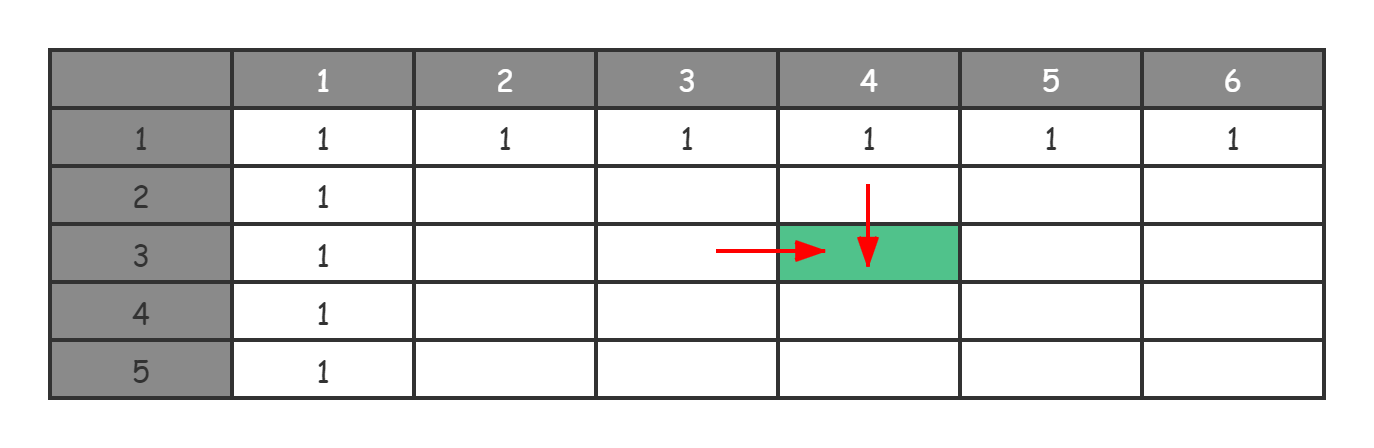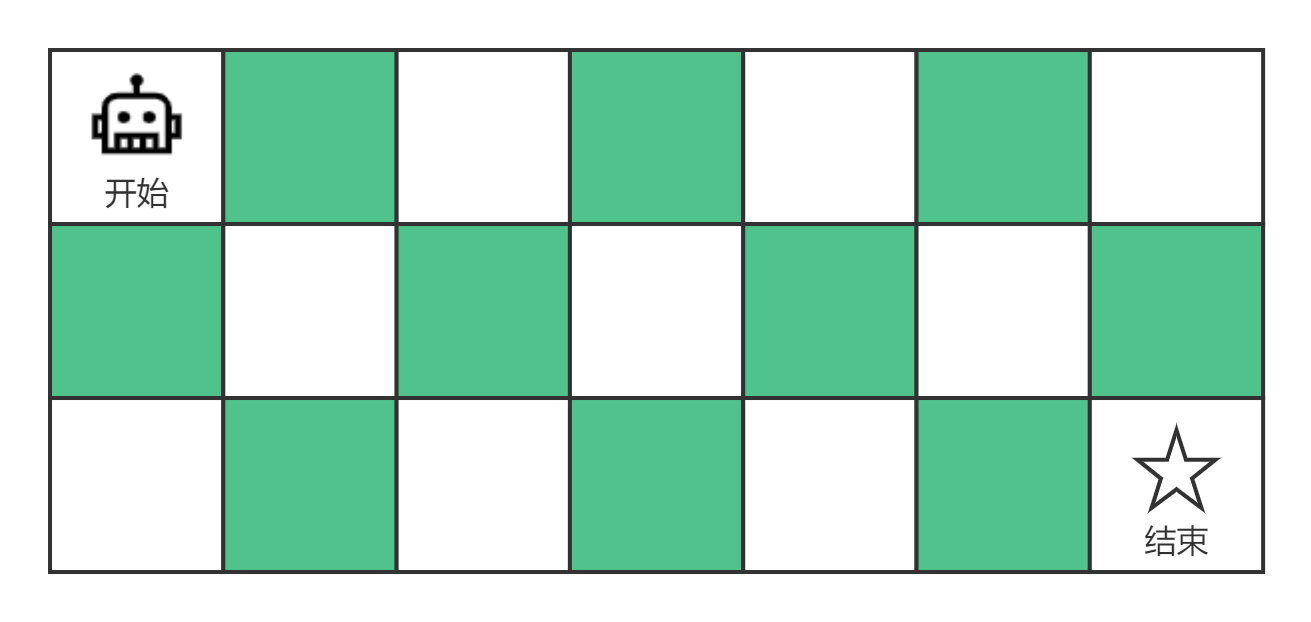10 面试即正义第二期：常见的动态规划面试题串烧

## 简单的路径规划

### 算法问题分析示例：

1. 向右 -> 向右 -> 向下
2. 向右 -> 向下 -> 向右
3. 向下 -> 向右 -> 向右


1. 重叠子问题：显然，求方案总数必定涉及穷举，那么在穷举过程中就难以避免出现重叠子问题的计算。比如说，格子 (4, 3) 的路径数量和格子 (3, 4) 的路径数量肯定都会依赖于格子 (3, 3) 的路径数量。因此，如果我们要求格子 (3, 3) 的路径数量，那么在求解格子 (4, 3) 和格子 (3, 4) 的时候，就不需要进行重复计算了；
2. 无后效性：然后判断该问题是否是无后效性的。由于我们的机器人只能向下或者向右走，因此格子的路径数量求解是单向的，所以子问题肯定是无后效性的；
3. 最优子结构：由于这个问题中机器人每次只能向右或者向下走一步，因此如果要产生不同的路径肯定从当前格子的上方下来，或者从当前格子的左侧过来，所以第 (m, n) 个格子的路径数量就是第 (m-1, n) 个格子的路径数量加上第 (m, n-1) 个格子的路径数量，所以这里存在所谓的最优子结构。

### 写出状态转移方程$DP(i, j)=\\left\\{\\begin{array}{c}- DP\[i-1$$j$ + DP$i$$j-1$, & if \\ i\\ne0\\ or\\ j\\ne0 \\\\\\- 1, & i=0\\ and\\ j=0- \\end{array}\\right.\]

### 编写代码进行求解

Java 实现：

int getPathCount(int m, int n) {
int[][] dp = new int[m][n];

// 初始化状态
for (int i = 0; i < m; i ++) { dp[i] = 1; }
for (int j = 0; j < n; j ++) { dp[j] = 1; }

for (int i = 1; i < m; i ++) { // 状态转移过程
for (int j = 1; j < n; j ++) {
dp[i][j] = dp[i - 1][j] + dp[i][j - 1];
}
}

return dp[m - 1][n - 1]; // 输出答案
}


C++ 实现：

int GetPathCount(int m, int n) {
int dp[m][n];

// 初始化状态
for (int i = 0; i < m; i ++) { dp[i] = 1; }
for (int j = 0; j < n; j ++) { dp[j] = 1; }

for (int i = 1; i < m; i ++) { // 状态转移过程
for (int j = 1; j < n; j ++) {
dp[i][j] = dp[i - 1][j] + dp[i][j - 1];
}
}

return dp[m - 1][n - 1]; // 输出答案
}


## 带障碍的路径规划

### 算法问题分析示例：

[
[0, 0, 0],
[0, 1, 0],
[0, 0, 0]
]

1. 向右 -> 向右 -> 向下 -> 向下
2. 向下 -> 向下 -> 向右 -> 向右


### 写出状态转移方程

$DP(i, j)=\\left\\{\\begin{array}{c}- 1, & i=0\\ and\\ j=0\\ and\\ u(i,j)=0 \\\\\\- 0, & u(i,j)=1 \\\\\\- DP\[i-1$$j$ + DP$i$$j-1$ & otherwise- \\end{array}\\right.\]

### 编写代码进行求解

Java实现：

int getPathCountWithBlocks(int[][] v) {
int m = v.length;
int n = v.length;

int[][] dp = new int[m][n];

// 初始化状态
for (int i = 0; i < m; i ++) { dp[i] = v[i] == 1 ? 0 : 1; }
for (int j = 0; j < n; j ++) { dp[j] = v[j] == 1 ? 0 : 1; }

for (int i = 1; i < m; i ++) { // 状态转移过程
for (int j = 1; j < n; j ++) {
if (v[i][j] == 1) {
dp[i][j] = 0;
} else {
dp[i][j] = dp[i - 1][j] + dp[i][j - 1];
}
}
}

return dp[m - 1][n - 1]; // 输出答案
}


C++实现：

int GetPathCount(const vector<vector<int>>& v) {
int m = v.size();
int n = v.size();

int dp[m][n]; memset(dp, 0, sizeof(dp));

// 初始化状态
for (int i = 0; i < m; i ++) { dp[i] = v[i] ? 0 : 1; }
for (int j = 0; j < n; j ++) { dp[j] = v[j] ? 0 : 1; }

for (int i = 1; i < m; i ++) { // 状态转移过程
for (int j = 1; j < n; j ++) {
if (v[i][j]) {
dp[i][j] = 0;
} else {
dp[i][j] = dp[i - 1][j] + dp[i][j - 1];
}
}
}

return dp[m - 1][n - 1]; // 输出答案
}


## 跳跃游戏

示例1：


示例2：



### 算法问题分析

1. 重叠子问题：这个问题肯定存在重叠子问题，比如求能否到达 $$i$$$$i-1$$ 这两个位置，那么肯定都需要确定 $$i-2$$ 这个位置的答案。因此，必定存在重叠子问题；
2. 无后效性：该问题明显也无后效性，只有后续的子问题依赖于前面的子问题；
3. 最优子结构：该问题是否存在最优子结构呢？当我们在一个位置的时候，我们可以知道最远可以跳到什么位置。因此，如果我们想要知道能否到达位置 $$i$$，就需要逐个看前面的位置，判定能否从 $$i-1$$$$i-2$$$$i-3$$ … 的位置跳到位置 $$i$$ 上。然后，再看 $$i-1$$ 这个位置是否能够到达，因此肯定存在最优子结构。

### 写出状态转移方程

$DP\[i$=\\left\\{\\begin{array}{c}- True, & i = 0 \\\\\\- (DP$j$ = true)\\ and\\ (max(A$j$+j) \\geq i), & i \\ne 0\\ and\\ j < i \\\\\\- \\end{array}\\right.\]

### 编写代码进行求解

Java 实现：

public boolean canJump(int[] nums) {
int n = nums.length;
if (n <= 1) { return true; }

boolean[] dp = new boolean[n];
// 初始化状态
for (int i = 0; i < n; i++) { dp[i] = false; }
dp = true;

for (int i = 1; i < n; i++) {
for (int j = 0; j < i; j++) { // j < i
if (dp[j] && j + nums[j] >= i) {
dp[i] = true;
break;
}
}
}

return dp[n - 1]; // 输出答案
}


C++ 实现：

bool canJump(vector<int>& nums) {
int n = nums.size();
if (n <= 1) { return true; }

bool dp[n]; memset(dp, 0, sizeof(dp));
dp = true; // 初始化状态

for (int i = 1; i < n; i++) {
for (int j = 0; j < i; j++) { // j < i
if (dp[j] && j + nums[j] >= i) {
dp[i] = true;
break;
}
}
}

return dp[n - 1]; // 输出答案
}